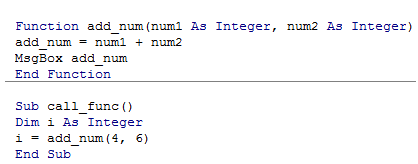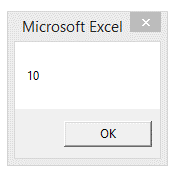## VBA functions in Excel

A function is also a piece of code tantamount to a subroutine, which performs some specific task. A function is called from a subroutine or another function.

A function can also be invoked at various part of the program similar to the subroutine

If everything is similar to a subroutine, then why use a function in excel VBA?

The fact is that, when you call a VBA function, you can send within the call of this VBA function some values or parameters and the VBA function will return a value or a set of values. Functions can return value or result unlike a subroutine which cannot return any values.

So, Subroutines are useful when you want to simply perform a certain task, but what if the resultant value of that task is needed by the calling function? This is where function comes to help.

For example you could create a VBA function that when you send a number between 1 and 26, could return the letter of the alphabet to which this number correspond.

Syntax

1. Function func_name (args)
2. /code
3. End Function

For a function to return a value, the following two conditons must be satisfied.

1.The datatype of the value to be returned must be declared in the function header.

2.The variable holding the return value must have the same name as that of function.

This variable does not need to be declared.

The following example illustrates this

1. Function add_num(num1 As Integer, num2 As Integer)
2. add_num = num1 + num2
4. End Function
5. Sub call_func()
6. Dim i As Integer
8. End Sub

A look at the code editor is as follows:And the output:Observe that, the calculated value from the function is returned back to the sub and stored in the variable i.